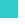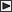BRI Research Paper

No.66

Numerical Simulation of Fire Phenomena and Its Application -Numerical Modeling of Fire Phenomena-.

Y.Hasemi; June, 1976. 32p.

Abstract

This paper presents a mathematical model of fire phenomena and its numerical solution method in consideration of chemical reactions, air movement and radiative heat transfer, and primarily consists of

 (1) Estimation method of radiative heat transfer in absorptive medium (2) Mathematical formulation of the behavior of strongly heated turbulent flow of air (3) Numerical calculation method of the non-linear difference equations for the behavior of compressible fluid flow by a finite difference methodLinksSitemapInquiryJapanese HP

BUILDING RESEARCH INSTITUTE, JAPAN